National Institute of Standards and Technology
 Computational Chemistry Comparison and Benchmark DataBase Release 20 August 2019 NIST Standard Reference Database 101
 I Introduction II Experimental data III Calculated data IV Data comparisons V Cost comparisons VI Input and output files VII Tutorials and Units VIII Links to other sites IX Feedback X Older CCCBDB versions XII Geometries XIII Vibrations XIV Reaction data XV Entropy data XVI Bibliographic data XVII Ion data XVIII Bad calculations XIX Index of properties XX H-bond dimers XXI Oddities

NIST policy on privacy, security, and accessibility.

The National Institute of Standards and Technology (NIST) is an agency of the U.S. Department of Commerce.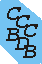# Tutorial on Electric Quadrupole Moments

## What is it?

Any distribution of charges can be described using a multipole expansion. The multipole expansion breaks the distribution down into the total charge, the dipole, the quadrupole, the octapole, etc.

## Practical considerations

Molecular electric quadrupole moments in general are derived (sometimes it seems in rather involved ways) from some other measured property. They are derived from the measurement of the second virial coefficient of gases (a measure of how non-ideal a gas is) and spectroscopic observations changes in the rotational energy levels when the molecule is in a magnetic or electric field. In both cases the quadrupole moment is a fudge factor that tweaks some other property. There is a physical basis for the expansion used in both cases, but I would not expect the values obtained from two such different measurements, with different random and systematic errors, to agree within the stated uncertainties of each measurement.

## Primitive? Traceless?

Some of the confusion over factors of two, and "primitive" versus "traceless" comes from this use of the quadrupole moment as a coefficient in different expansions describing different properties. The electric quadrupole moment can be described as a 3x3 symmetric matrix with components:

 Qxx Qxy Qxz Qxy Qyy Qyz Qxz Qyz Qzz
This has 6 different values. However, the traceless version is used to omit the spherical component. An experimental measurement will observe the part of the quadrupole that is different from spherical. Just to make it a little more confusing instead of just subtracting the trace the diagonal elements of the "traceless" matrix are defined as:
xx' = (3*xx-trace)/2
yy' = (3*yy-trace)/2
zz' = (3*zz-trace)/2
I believe this comes from the use of the components in particular equations.

One reference that discusses how the "gas-property people" use the quadrupole is in: "Theory of Molecular Fluids Volume1: Fundamentals" C.G. Gray and K.E. Gubbins, Oxford University Press, New York, 1984

## Relation between cartesian and spherical components

monopole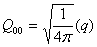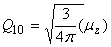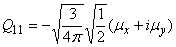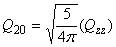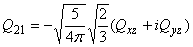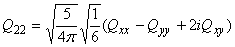However one often wants just the magnitude of the quadrupole, where the magnitude of a general multipole moment is defined as: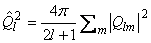The previous equations are from "Theory of Molecular Fluids Volume1: Fundamentals" C.G. Gray and K.E. Gubbins, Oxford University Press, New York, 1984